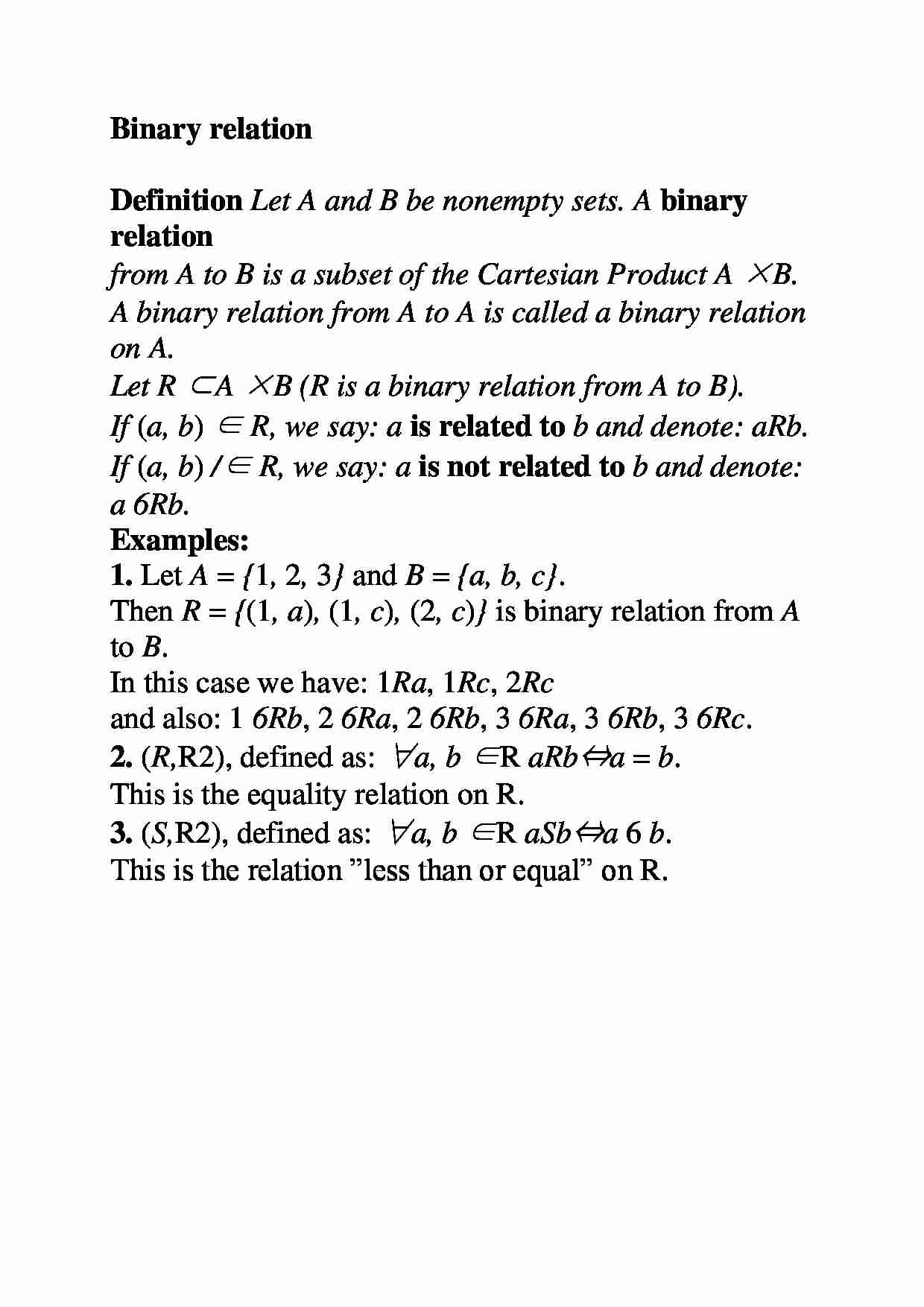# Relacja Binar

Nasza ocena:

Wyświetleń: 854
Komentarze: 0

### Pobierz ten dokument za darmo

Podgląd dokumentu## Fragment notatki:

Binary relation Definition Let A and B be nonempty sets. A binary relation from A to B is a subset of the Cartesian Product A × B . A binary relation from A to A is called a binary relation on A . Let R ⊂ A × B ( R is a binary relation from A to B ). If ( a, b ) ∈ R , we say: a is related to b and denote: a R b . If ( a, b ) / ∈ R , we say: a is not related to b and denote: a 6R b . Examples: 1. Let A = { 1 , 2 , 3 } and B = { a, b, c } . Then R = { (1 , a ) , (1 , c ) , (2 , c ) } is binary relation from A to B . In this case we have: 1 R a , 1 R c , 2 R c and also: 1 6R b , 2 6R a , 2 6R b , 3 6R a , 3 6R b , 3 6R c . 2. ( R , R 2 ) , defined as: ∀ a, b ∈ R a R b ⇔ a = b . This is the equality relation on R . 3. ( S , R 2 ) , defined as: ∀ a, b ∈ R a S b ⇔ a 6 b . This is the relation ”less than or equal” on R . ... zobacz całą notatkę

## Komentarze użytkowników (0)

Zaloguj się, aby dodać komentarz